### IMO Shortlist 1999 problem N4

Kvaliteta:
Avg: 0,0
Težina:
Avg: 7,0
Denote by S the set of all primes such the decimal representation of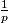$\frac{1}{p}$ has the fundamental period divisible by 3. For every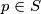$p \in S$ such that$\frac{1}{p}$ has the fundamental period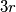$3r$ one may write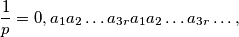where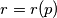$r=r(p)$; for every$p \in S$ and every integer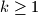$k \geq 1$ define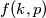$f(k,p)$ by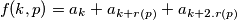a) Prove that$S$ is infinite.
b) Find the highest value of$f(k,p)$ for$k \geq 1$ and$p \in S$
Izvor: Međunarodna matematička olimpijada, shortlist 1999